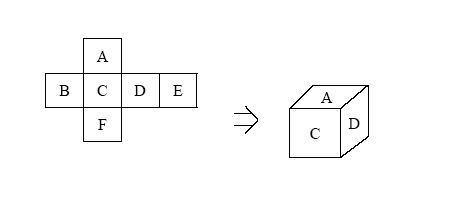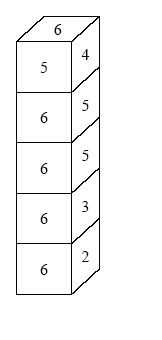Online JudgeProblem SetAuthorsOnline ContestsUser
Web Board
F.A.Qs
Statistical Charts
Problems
Submit Problem
Online Status
Prob.ID:
Register
Authors ranklist
Current Contest
Past Contests
Scheduled Contests
Award Contest
Register
Language:
Dice Stacking
 Time Limit: 1000MS Memory Limit: 30000K Total Submissions: 1486 Accepted: 905

Description

Chun-Soo is playing a dice stacking game. Six faces of a die are squares of the same size; each face of a die has a number from 1 to 6. But, they are not standard dice because the sum of the numbers in the opposite faces may not always be 7.

The dice game is to stack several dice up in the order Die 1, Die 2, Die 3, from the base keeping the following rule: For each pair of consecutive dice, the number on the bottom of the top die must match the number on the top of the bottom die. In other words, the two faces that are together must have the same number. We are free to place the bottom die as we wish, so Die 1 can be set freely.

A long rectangular pillar which has 4 sides is created. We are trying to make one side have the maximum sum. (Note that after we rotate a die to fix the top face and bottom face, we can still rotate the die by 90, 180, or 270 degrees.) Write a program to find the maximum sum that one side can have.

Input

The first line of the input contains a single integer t (1 <= t <= 11), the number of test cases, followed by the input data for each test case. The first line for each test case contains an integer n (1 <= n <= 10,000), the number of dice. In the next lines, each line contains six integers for a die, by the order A, B, C, D, E, F, as in the following figure. There is a single space between two numbers. The number of dice is less than or equal to 10,000. It is possible for two dice to be the same.Output

There should be one line per test case which contains the maximum sum of the numbers in one side.

Sample Input

```1
5
2 3 1 6 5 4
3 1 2 4 6 5
5 6 4 1 3 2
1 3 6 2 4 5
4 1 6 5 2 3
```

Sample Output

`29`

Hint

To get the maximum sum for the above input, you should pile up the dice as follows:Source

[Submit]   [Go Back]   [Status]   [Discuss]Home PageGo BackTo top